# 10. The planimeter: the instrument that measures natural selection and Darwinian evolution

Since this is science, we must eventually provide replicable measures for natural selection and Darwinian evolution. The instrument we use must not only be reliable; and not only must we demonstrate that it is capable of measuring the properties we stipulate; we must additionally prove that those stipulated properties are representative of natural selection and of Darwinian evolution. We must also of course correct any perceived misconceptions within biology and ecology about basic scientific terms, as well as determine biological equivalents for mass, inertia and so forth.

If we truly want to emulate Newton and produce some similar laws for Darwin’s natural selection, and for the biology and ecology that depend upon it, then we certainly need some vectors. Vectors mean we must be able to measure in different directions upon orthogonal axes if needed. We must relate those measures to each other. The orthogonal axes needed take us into geometry, areas, rectangles and parallelograms.

By a happy coincidence, the instrument we shall use was used by Darwin himself as he prepared to write his Insectivorous Plants. He studied carnivorous plants with a view to determining what adaptations might allow them to live when conditions were so difficult that they had to seek for animal nutrients (Darwin, 1875). He concluded that adaptations such as sticky fluids to ensnare prey were an example of natural selection pressure:

My son Francis counted the hairs on a space measured by means of a micrometer, and found that there were 35,336 on a square inch of the upper surface of a leaf, and 30,035 on the lower surface; that is, in about the proportion of 100 on the upper to 85 on the lower surface. … A moderately fine plant bearing twelve leaves (the larger ones being a little more than 2 inches in diameter) was now selected, and the area of all the leaves, together with their foot-stalk (the flower-stems not being included), was found by a planimeter to be 39.285 square inches; so that the area of both surfaces was 78.57 square inches (Darwin, 1875).

Figure 6: Equivalence of Rectangle and Parallelogram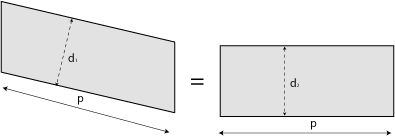Figure 7: Rectangular Addition and Subtraction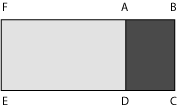Figure 6 reminds us that for every parallelogram, there exists a rectangle that matches its area. Therefore, once the base and height of any parallelogram’s equivalence-rectangle is known, we know that given parallelogram’s area. Figure 7 is a gentle reminder that rectangles are extensive: if we remove rectangle FADE from FBCE, we are left with area ABCD.

We should also carefully note that we can determine all these dimensional areas simply by knowing their boundaries—i.e. by knowing chosen one-dimensional length measures along their various perimeters. That is to say, if we can find two suitable orthogonal axes, then we can determine what is contained within any bounded figure located between those axes in terms of the lengths of the containing boundary.

Planimeters were first invented in 1818 by Johann Martin Hermann, a Bavarian land surveyor (Care, 2006). His contained the core essentials: a cone and wheel mounted on a track. Darwin was an early adopter. They are still used in some branches of ecology, but remain relatively unknown. Richard Hauer & Gary Anthony Lamberti’s Methods in stream ecology and Peter Greig-Smith’s Quantitative plant ecology describe some uses. David Murray Gates and others used them for measuring the wing areas of insects and birds, while Gist and Swank describe a further usage in their “An Optical Planimeter for Leaf Area Determination” (Hauer & Lamberti, 2007; Greig-Smith, 1984; Gates and Schmerl, 1975; Gist & Swank, 1974). Their biological and ecological provenance is thus beyond dispute. Their big advantage is the link they provide between a boundary and an area—between a given object’s surround, and its content. Since they are essential to the development of this vector-based argument, we shall take a quick look at how they work (Encyclopaedia Britannica 1911; Leise, 2007; Kunkel, 2008; Eggers, 2010; Skinner, 2010; Rogers).

Figure 8: Planimeter & Rectangle (1)Figure 8 shows a linear planimeter being used to determine the area of an arbitrary rectangle, ABCD. We have established our two axes, with one stretching along Y. The planimeter’s rod, of known length p, stretches along Y from P1 to P2. On the rod is a wheel, W, suitably calibrated to record all its movements, of whatever variety, relative to the two axes. A pin attaches to P2, which allows any boundary to be traced. As the pin at P2 is moved about the boundary of the object being traced, the wheel’s movements are always normal to the rod, while its end P1 always moves vertically up and down Y. The angle between Y and the wheel will of course change with any of P2’s horizontal movements as the given boundary is traced..

If we now use our planimeter to trace downwards from A to B in Figure 8, the rod will maintain a constant given angle to Y, and the planimeter will sweep out a parallelogram of known dimensions. P1 will move from Y1 to Y2. The wheel moves normally through the distance d1. The ratio between d1 and Y1-Y2 ( = AB) depends upon the angle the rod moves with respect to Y. But since we have the normal, the parallelogram’s area is pd1. We can now calibrate our planimeter so that any given linear reading, d, can be scaled up by a given constant—say Z, and which depends entirely upon the planimeter’s physical dimensions—to produce the area it has swept out.

Figure 9: Planimeter & Rectangle (2)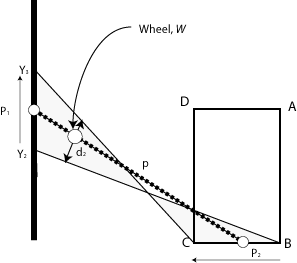We may have thus far produced a parallelogram’s area, but it is not the rectangle ABCD we are seeking. We therefore proceed, as in Figure 9, to move P2 along BC. The wheel will now move sideways, further away from Y, as the end P1 is now forced to move upwards from Y2 to Y3. But the angle between the rod and its axis is also changing by a set amount. The wheel will record the distance d2. We produce the two triangles shown, and whose areas are in principle determinable from those angles and the distance d2. The wheel has now therefore moved a total distance D = d1 + d2. If we now apply our planimeter constant Z and so relate it to the amount the rod has both travelled and rotated, then D will give us the area sum of both the parallelogram and the two triangles.

Figure 10: Planimeter & Rectangle (3)Figure 10 now sees us tracing side CD of our rectangle … and in a direction in opposition to the AB we started with. Our planimeter is therefore sensitive to direction. This is going to help us with any vectors we might meet. We move higher up Y, from Y3 to Y4, and the wheel measures out d3. It produces a second parallelogram whose area is pd3. But since P2 is closer to the axis, so making the angle greater than when we measured d1 for AB, the resulting parallelogram’s area is smaller. And since we are moving in an opposite direction, then the distance measured by the wheel is negative and subtracts itself from the total value. The total distance now travelled is thus D = d1 + d2 - d3. We added the first parallelogram’s area … but we have now removed the second. Unfortunately, we still have the two triangles from d2 and BC in play, but that problem is soon solved.

Our final journey (not depicted) is now DA. We will now descend on Y from Y4 back to Y1 where we began. Since this is in opposition to our previous horizontal—and complementary—excursion along BC, then the total distance the wheel travels will be D = d1 + d2 - d3 - d4, because d2 will be opposed to d4. These two horizontal journeys are exact reverses because we will eventually subtend our original angle between rod and axis. By the doctrine of similar triangles these two complementary journeys will produce triangle-couples whose areas are identical. One triangle in each pair is not just similar to the other but congruent. And since d4 = d2 then these two triangle-sets cancel out, and the total wheel distance is D = d1 - d3 … which is now the area of our target rectangle, ABCD. It is simply the difference in the areas of the two rectangles that represent the two parallelograms produced by tracing its boundary. When we apply our constant, Z, to the value on our dial, we have the value we seek. A one-dimensional vector reckoning along a boundary produces a two-dimensional area.

Figure 11: Planimeter and Curved Area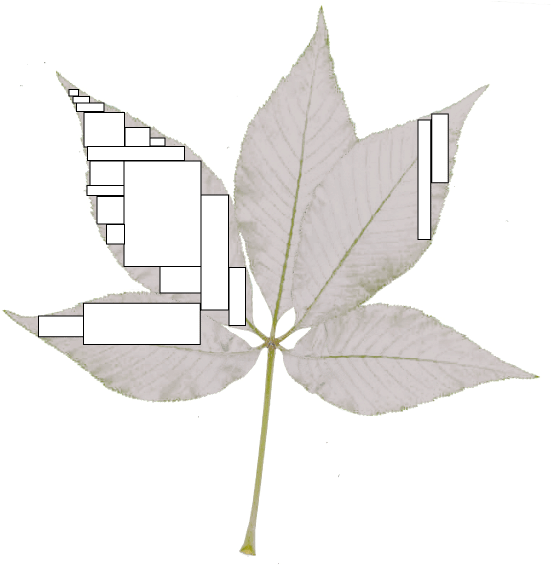Since we can now determine the area of any arbitrary rectangle, we can divide any equally arbitrary shape—such as the leaf in Figure 11—into a collection of rectangles. We can then trace around their respective boundaries and determine an area. But … this now enters the realms of the integral and differential calculus for the greater is the number of such rectangles, then the better is the approximation, and the more accurately do we know the area.

Figure 12: Planimeter: Green's Theorem in the Plane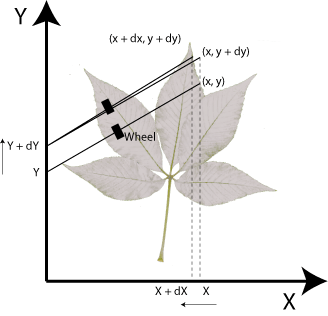As in Figure 12, we can now instead consider our leaf to be surrounded by two orthogonal axes, X and Y. As the tracer arm moves between the two locations in the figure, the rod and wheel move the two distances dx and dy respectively, and so from x and y to (x + dx) and (y + dy) again respectively. Since the wheel pivots, it moves through the infinitesimal angle dθ to produce all those values in the x component. The wheel’s x movements are always available through the respective sines and cosines. But since, if we go all the way around the leaf, θ = 360° = 0°, then all those movements in x—i.e. the entire set of transverse movements—can be ignored because they inevitably cancel out in the same way the triangle couples did. We are thus left with the net sums in y as our result.

And … this now takes us directly to the vector calculus and to Green’s theorem, one of its four fundamental theorems. By Green’s theorem, if a curve, C, is closed, positively oriented, and piecewise smooth such that it bounds a simply connected region R, and on which the functions F1(x, y) and F2(x, y) each have continuous partial derivatives, then:

C F1 dx + F2 dy = R ((∂F2/x) - (∂F1/y))  dA.

The line integral upon the left produces the bounded area of the surface integral R on the right for a very large number of choices of values for F1 and F2. Since our planimeter’s movements in y can be reckoned invariant while x moves and conversely, we can declare both F1 = 0, F2 x and F1 = -y, F2 = 0. This then gives a simpler validation for our linear planimeter. As long as we close the curve and complete our circuit, then all movements of x along y can be taken, while all those of y along x are removed because they cancel. Whatever value results is then the area concerned as in:

C x dy +C y dx =R   dA = value for area of R.

Green’s theorem also gives us access to the behaviours of any conceivable vector phenomenon or field. We simply divide that force into two components. We allocate a first component, F1 to the x-axis, and a second component, F2 along the y-axis. Our force, F, is then described by F1F2j; and where T is the unit tangent vector; and in which a circulation in F exists all around R as our vector that moves relatively to these two components. We then have:

C  F • T ds = C F1 dx + F2 dy.

Green’s theorem is widely used in molecular biology and biophysics and performs for us an important service. Leon and Roberge, for example, describe its usage in A new cable model formulation based on Green’s theorem where they develop equations describing the potentials either side of cellular membranes; and Michael Altman et al use it to describe An Accurate Surface Formulation for Biomolecule Electrostatics in Non-Ionic Solutions (Leon and Roberge, 1990; Altman et al, 2005). It has also been used to measure phenomena as disparate as the cross-sections of tumours, and the areas of forests. Green’s theorem therefore gives us access to molecules as they both exert and respond to forces—Newtonian vector forces—and that do work and receive and expend energy at the molecular levels … and so therefore at the macroscopic ones created by those molecular movements in force and with energy.

In other words, our planimeter, in recording the combinations of distances travelled on a boundary, and in orthogonal coordinates x and y, now tells us everything we need to know about any phenomenon described in two dimensions, and all simply by knowing its value, as summed or integrated, about that boundary. This will certainly allow us to measure natural selection and Darwinian evolution … if we can describe them suitably. The journey to do so is a little circuitous, but well worth the effort. We end up with a set of differential equations that both describe the entirety of biology and establish a quantum biology.